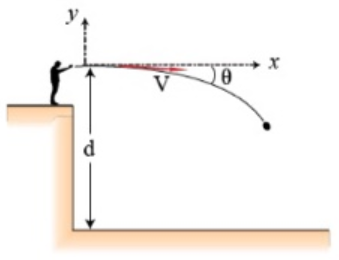# Problem: A student standing on a cliff that is a vertical height = 8.0 m above the level ground throws a stone with velocity Vo = 25 m/s at an angle = 22° below horizontal. The stone moves without air resistance: use a Cartesian coordinate system with the origin at the stone's initial position. Part (a) With what speed, vf in meters per second, does the stone strike the ground?

###### FREE Expert Solution

In this problem, we'll use the kinematic equation:

$\overline{){{{\mathbit{v}}}_{{\mathbit{f}}}}^{{\mathbf{2}}}{\mathbf{=}}{{{\mathbit{v}}}_{{\mathbf{0}}}}^{{\mathbf{2}}}{\mathbf{-}}{\mathbf{2}}{\mathbit{g}}{\mathbf{∆}}{\mathbit{y}}}$

88% (315 ratings)###### Problem Details

A student standing on a cliff that is a vertical height = 8.0 m above the level ground throws a stone with velocity Vo = 25 m/s at an angle = 22° below horizontal. The stone moves without air resistance: use a Cartesian coordinate system with the origin at the stone's initial position.Part (a) With what speed, vf in meters per second, does the stone strike the ground?

Frequently Asked Questions

What scientific concept do you need to know in order to solve this problem?

Our tutors have indicated that to solve this problem you will need to apply the Projectile Motion: Horizontal & Negative Launch concept. If you need more Projectile Motion: Horizontal & Negative Launch practice, you can also practice Projectile Motion: Horizontal & Negative Launch practice problems.

What professor is this problem relevant for?

Based on our data, we think this problem is relevant for Professor Jiang's class at USF.